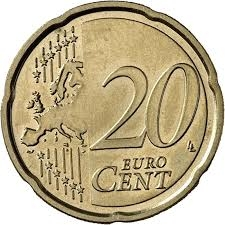# Exchanged 18573

The merchant exchanged CZK 1,000 (CZK is the Czech crown) for twenty and ten crowns in the bank. He had a total of 70 coins. How many crowns did he have? How many crowns did he have?

a =  40
b =  30

### Step-by-step explanation:

a+b=70
1000 = 10a+20b

a+b=70
1000 = 10·a+20·b

a+b = 70
10a+20b = 1000

Pivot: Row 1 ↔ Row 2
10a+20b = 1000
a+b = 70

Row 2 - 1/10 · Row 1 → Row 2
10a+20b = 1000
-b = -30

b = -30/-1 = 30
a = 1000-20b/10 = 1000-20 · 30/10 = 40

a = 40
b = 30

Our linear equations calculator calculates it.Did you find an error or inaccuracy? Feel free to write us. Thank you!

Tips for related online calculators
Do you have a system of equations and looking for calculator system of linear equations?